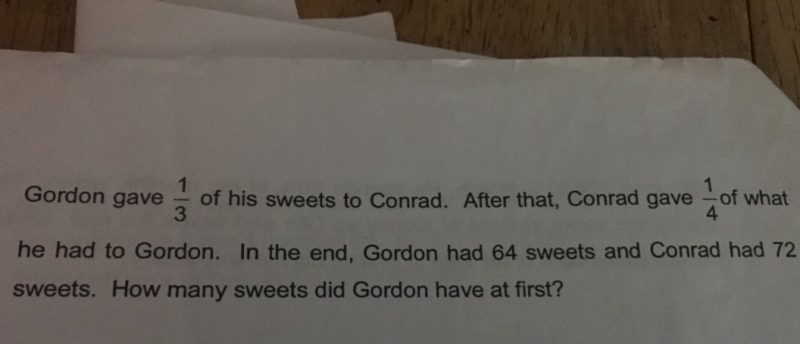# QuestionHi,

Thank you

Therefore, 3/4 of Conrad sweets = 72

1/4 of Conrad sweets = 72/3 = 24

So, 64 – 24 = 40 sweets

This 40 sweets is 2/3 of what he had at first because he gave 1/3 to Conrad.

So, 1/3 = 40/2 = 20

3/3 = 20*3 = 60 sweets

Steps